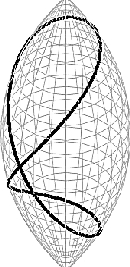# Open problems in systolic geometry and topologyThere are currently over 300 systolic papers on the arXiv

Systolic geometry in MathSciNet (Math Reviews)

A. Problems on surfaces:

A1. there are various asymptotic constants that can be defined for surfaces of large genus. The asymptotic behavior is rather well understood but the optimal asymptotic constants are not known. Here arithmeticity and ergodic ideas play an important role.

A2. Are all aspherical surfaces, Loewner?

A3. do systolic loops of an extremal surface generate the homology? Do almost systolic loops (=loops of length at most twice the systole) of an almost extremal surface generate the homology?

A4. Several norms can be defined on the real homology space of a Riemannian surface, for instance the stable norm and the L^2 norm. Consider the Jacobi embedding from the surface into the Jacobi torus defined as the quotient of the real homology space by the integral homology lattice. Determine the systole of the surface for the pull-back Finsler metric, especially its asymptotic behaviour for large genus.

B. Problems on the relation between systolic geometry and topology:

B1. Gromov's proof of the systolic inequality for essential manifolds relied on filling invariants. Following Larry Guth's recent article in GAFA exploiting cup-products, develop a filling-free proof of the inequality, in as great a generality as possible.

B2. Back to filling radius: strengthen (1) to a lower bound for the volume of a ball of radius less than FillRad. This has been proved by Guth (to appear in Annals of Mathematics).

B3. Develop finer 1-systolic bounds, say in the framework of n-tori. This would involve something similar to the successive minima of the integer lattice in homology with respect to the stable norm, in the case of the stable 1-systole.

B4. clarify the situation with the stable systolic category of M. There was a paper by Brunnbauer, and a sequel by Dranishnikov-Rudyak, but the general picture is not completely clear, particularly in relation to rational cup length of M (this already connects to the next section).

C. Problems on higher systoles (k-systoles):

C1. there is the intriguing problem of the optimal stable 4-systolic ratio of 8-manifolds, say with unit b_4: b_4(M)=1. Here it turns out that the ratio is more or less independent of the manifold. The standard metric on CP^4 has a better ratio than the standard metric on HP^2, which shows that the latter is not optimal. Yet the best value is not known and may be related to the Cayley 4-form, E_7, Joyce manifolds, etc (see recent reprint). A similar question for CaP^2 seems hopeless at this point.

C2. Systolic geometry of coverings: find good bounds on the k-(stable)-systole of covering towers of a manifold with a fixed metric (say a locally symmetric space), especially bounds that can detect the rank of the manifold.

C3. Find a uniform lower bound for the volume of an n-manifold satisfying a suitable condition on its cup product in terms of its k-stable-systole. Thus, the volume of an orientable 4-manifold with nonzero b_2 is always bounded from below by C(b_2) stsys_2(M)^2 (since the intersection pairing in middle dimension is nontrivial by Poicare duality), but the constant C(b_2) decreases with b_2.

C4. More generally, find systolic inequalities which take into account the topology of the manifold and get better when the topology is "complicated". For instance, are there finitely many groups which can arise as the fundamental group of a closed aspherical manifold with bounded systolic volume ?

C5. (Michael Freedman) When there is systolic freedom, one can try to quantify it. Say restrict to metrics with curv < 1, inj rad > 1, and volume V, and look at the systolic ratio and see how it grows with V. Freedman points out that his mod 2 systolic freedom examples (in dimension 3) only grow like log V, and he raised the question what is the real rate of growth. The question seems interesting for all systolic ratios.

C6. Questions about k-dilations (stretching of k-dimensional areas. There are a few questions about k-dil and systoles, such as the following. Let g_0 be the product of unit sphere metrics on S^n x S^n. Fix k > n, and suppose that g has all k-dimensional areas bounded by those in g_0. For k = 2n this implies that total volume of g is less than than of g_0 and nothing else, but for smaller k it becomes a much stronger condition. For such g, can one bound Sys_n(g)?

C7. One knows that the k-systole is unbounded on the space of all metrics of volume one on a given manifold for k>1. Determine the local maxima of the k-systole. Gromov showed that the normalized Fubini-Study metric on CP^2 is a local maximum for the 2-systole, but this is the only known example in dimension greater than two.

D. A question contributed by Gromov:

What are the Riemannian manifolds which are extremal for (curvature free) metric inequalities?

The known examples are:

0. rectangular solids in R^n for the Besicovich inequality.

1. projective plane, the 2-torus, and the Klein bottle for the systolic inequalities.

2. Burago-Ivanov inequality for the asymptotic volume growth of coverings of tori.

3. The symmetric spaces of non-compact type for the entropy-volume inequalities by Katok and Besson-Gallot-Cortois.

4. Domains in the hyperbolic plane for filling area inequality.

Specifically, which manifolds of constant curvature (with or without) boundary are extremal for suitable problems? For example, is RP^n systolically extremal? Do hemisheres minimize filling volume and or radius?

E. If you would like to contribute other questions, conjectures, or corrections, please write to Guth, Sabourau, or Katz.

F. Further open problems may be found in Guth's "Metaphors" text, see Larry Guth